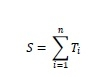# C/C++ Program to Find sum of Series with n-th term as n power of 2 - (n-1) power of 2

Here we will see how to get the sum of the series with n-th term as n2 – (n-1)2. The recurrence relation is like below −

Tn = n2 − (n−1)2

So the series is −We need to find S mod (109 + 7), where S is the sum of all terms of the given series.

## Example

#include<iostream>
#define X 1000000007
using namespace std;
long long getSum(long long n) {
return ((n % X) * (n % X)) % X;
}
int main() {
long long n = 56789;
cout << getSum(n);
}

## Output

224990500